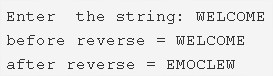# C Program To Find Reverse Of A string | 4 Ways

C program to find the reverse of a string – In this article, we will discuss the several methods used to find the reverse of a string in C programming.

Suitable examples and sample programs have also been added so that you can understand the whole thing very clearly. The compiler has also been added with which you can execute it yourself.

The methods used in this piece are as follows:

• Using Standard Method
• Using Function
• Using Recursion
• Using String Library Function

A string is nothing but an array of characters. The value of a string is determined by the terminating character. Its value is considered to be 0.As you can see, you need to enter a string of your own choice firstly.

The string entered here is a simple “WELCOME”.

The string that will emerge after it is reversed is this:

EMOCLEW

Thus, the means to do so in C programming are as follows:

## Using Standard Method

1. Read the entered string and store the string into the variable s.

2) Calculate the string length using strlen(String s) and store into the variable n.

3) Iterate the for loop from i=0 to i<half of the length of the string.

a) Initialize the starting element to temp.

b) Initialize the s[n-i-1] element to s[i].Here last positioned character initialized to the first position.

c) Initialize the temp to last position.

when ever i value increased then the position will be decreased.

4) Print the reverse of a string.

Output:

### Using Function

1. The main() calls the stringreverse(char *s) function by passing the string as an argument to that function.

2) The function first calculates the string length using string library function strlen(String) and assign to n.

a) Iterate the for loop from i=0 to i<half of the length of the string.

Initialize the starting element s[i] to temp.

Initialize the character from the last position s[n-i-1] to starting position s[i].

Initialize the temp to last position s[n-i-1].

3) Print the reverse string.

Output:

#### Using Recursion

1. The function stringreverse(char *s) calculates the string length using strlen(s) and length will be stored in the variable n.

a) If i<length of the string/2

initialize the s[i] to temp

initialize element from last index n-i-1 to s[i] and temp to last index s[n-i-1].

i value increased by 1

the function calls itself recursively until i< half of the string length.

2)Print the reverse string.

Output:
##### Using String Library Function
1. The function strrev(String s) is the one of the library function of string. It will reverse the given string.

2) The function strrev(String s) is available in the string.h header file.

Output:
x

## C Program To Print Number Of Days In A Month | Java Tutoring

C program to input the month number and print the number of days in that ...